# User:Evil4Zerggin/Effective position drag model

The idea is to have an easily invertible function that maps the effective position---where the projectile would be if there were no drag---to the actual position of the projectile. Then to hit a target point, we simply invert the target coordinates and aim at that point instead.

## Basics

Let the function that maps the effective position of the projectile to the actual position be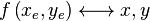$f \left( x_e, y_e \right) \longleftrightarrow x, y$

The trajectory of a dragless projectile is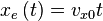$x_e \left( t \right) = v_{x0} t$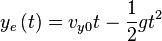$y_e \left( t \right) = v_{y0} t - \frac{1}{2} g t^2$

The trajectory of the projectile after drag is then$f \left( v_{x0} t, v_{y0} t - \frac{1}{2} g t^2 \right)$

To hit a point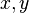$x, y$, we instead aim at$f^{-1} \left(x, y\right) \rightarrow x_e, y_e$

as if there were no drag.

## Choosing the function

Of course,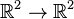$\mathbb{R}^2 \rightarrow \mathbb{R}^2$ is a very large space. Let us see what sort of functions might be appropriate.

•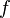$f$ should be continuous.
•$f$ doesn't necessarily have to be onto---it's fine if shells can't reach some parts of the world. But it should probably be one-to-one, as otherwise we will almost certainly suffer discontinuities in the inverse.
• One possibility for simplification is making$f$ depend on and/or affect only the distance from the origin. However, this could cause some strange trajectories with high-angle fire.
• Another is to separate the$x$ and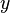$y$ directions so that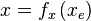$x = f_x \left(x_e\right)$ and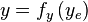$y = f_y \left(y_e\right)$.
• In fact, we may even consider dispensing with modifying$y$ at all, in which case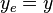$y_e = y$,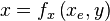$x = f_x \left(x_e, y \right)$, and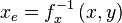$x_e = f_x^{-1} \left(x, y \right)$.
•$f$ could of course also depend on fixed properties of the projectile.
•$f$ should map the origin to the origin, and we should have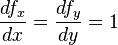$\frac{df_x}{dx} = \frac{df_y}{dy} = 1$ (no scaling near the origin).

### Some x-only possibilities

•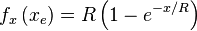$f_x \left( x_e \right) = R \left(1 - e^{-x / R} \right)$ for some maximum horizontal range parameter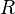$R$. This corresponds to linear drag in the horizontal axis only.
•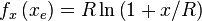$f_x \left( x_e \right) = R \ln \left(1 + x / R \right)$. This corresponds to quadratic drag in the horizontal axis only.

## Other considerations

• At the least additional work would be needed to deal with inherited velocity.
• Affecting only the horizontal axis will cause shells to plunge pretty heavily at the end. Though maybe that could be a feature. On the other hand, if they keep their vertical velocity in space, they will tend to curve upwards...